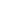# Problem: Which of these ions is the best reducing agent?  Standard Reduction Potentials                   E°      Fe 3+ (aq) + e– → Fe 2+ (aq)                + 0.77 V Cu 2+ (aq) + e– → Cu + (aq)                 + 0.15 V   a) Fe 3+ b) Fe 2+ c) Cu 2+ d) Cu +

###### FREE Expert Solution
80% (189 ratings)
###### Problem Details

Which of these ions is the best reducing agent?

Standard Reduction Potentials                   E°

Fe 3+ (aq) + e → Fe 2+ (aq)                + 0.77 V

Cu 2+ (aq) + e– → Cu + (aq)                 + 0.15 V

a) Fe 3+

b) Fe 2+

c) Cu 2+

d) Cu +What scientific concept do you need to know in order to solve this problem?

Our tutors have indicated that to solve this problem you will need to apply the Redox Reaction concept. You can view video lessons to learn Redox Reaction. Or if you need more Redox Reaction practice, you can also practice Redox Reaction practice problems.

What is the difficulty of this problem?

Our tutors rated the difficulty ofWhich of these ions is the best reducing agent?  Standard R...as low difficulty.

How long does this problem take to solve?

Our expert Chemistry tutor, Jules took 1 minute and 28 seconds to solve this problem. You can follow their steps in the video explanation above.

What professor is this problem relevant for?

Based on our data, we think this problem is relevant for Professor Shiraiwa's class at UCI.